# 4 Mass Spectrometry Objectives 1 Know what information

• Slides: 11
Download presentation4. Mass Spectrometry Objectives: 1. Know what information they can provide 2. Interpret simple mass spectra graphs 3. Know some uses of mass spectrometryA mass spectrometer…. • Draw the mass spectrometer • Describe the 5 step process AS A CARTOON STORY BOARD! • Extension: Use your own words to describe the stagesMass Spectrometry The first mass spectrometer was built in 1918 by Francis W Aston, a student of J J Thomson, the man who discovered the electron. Aston used the instrument to show that there were different forms of the same element. We now call these isotopes. In a mass spectrometer, particles are turned into positive ions, accelerated and then deflected by an electric or magnetic field. The resulting path of ions depends on their ‘mass to charge’ ratio (m/z). Particles with a large m/z value are deflected least those with a low m/z value are deflected most. Francis Aston The results produce a mass spectrum which portrays the different ions in order of their m/z value. USES Mass spectrometry was initially used to show the identity of isotopes. It is now used to calculate molecular masses and characterise new compounds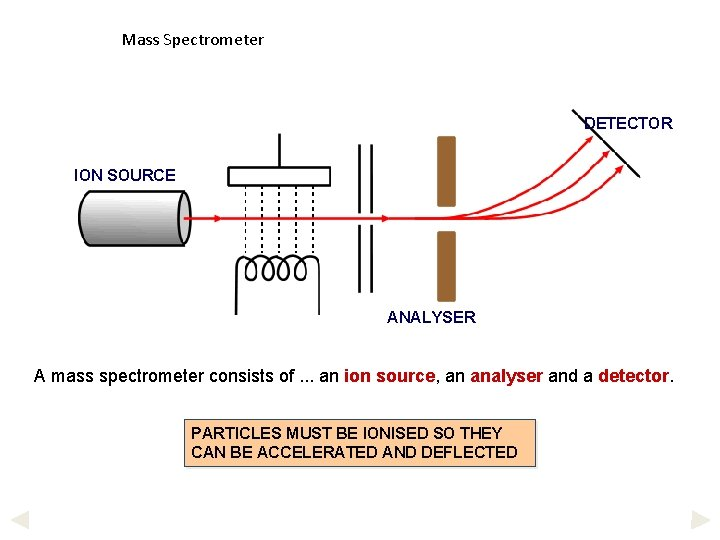Mass Spectrometer DETECTOR ION SOURCE ANALYSER A mass spectrometer consists of. . . an ion source, an analyser and a detector. PARTICLES MUST BE IONISED SO THEY CAN BE ACCELERATED AND DEFLECTEDHow does it work? DETECTOR ION SOURCE ANALYSER IONISATION • gaseous atoms are bombarded by electrons from an electron gun and are IONISED • sufficient energy is given to form ions of 1+ charge ACCELERATION • ions are charged so can be ACCELERATED by an electric field DEFLECTION • charged particles will be DEFLECTED by a magnetic or electric field DETECTION • by electric or photographic methods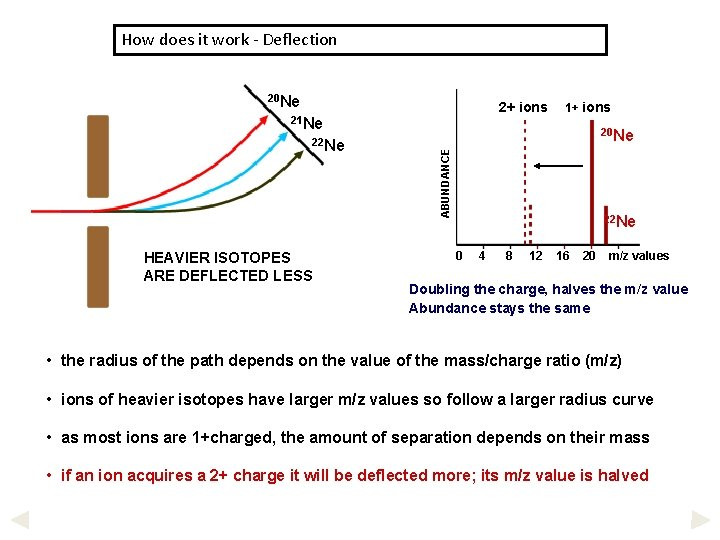How does it work - Deflection 20 Ne 2+ ions 21 Ne HEAVIER ISOTOPES ARE DEFLECTED LESS 20 Ne ABUNDANCE 22 Ne 1+ ions 22 Ne 0 4 8 12 16 20 m/z values Doubling the charge, halves the m/z value Abundance stays the same • the radius of the path depends on the value of the mass/charge ratio (m/z) • ions of heavier isotopes have larger m/z values so follow a larger radius curve • as most ions are 1+charged, the amount of separation depends on their mass • if an ion acquires a 2+ charge it will be deflected more; its m/z value is halvedWhat is a Mass Spectrum? 20 Ne MASS SPECTRUM OF NEON 90. 92% 21 Ne 0. 26% 22 Ne 19 20 21 22 8. 82% 23 In early research with a mass spectrograph, Aston (Nobel Prize, 1922) demonstrated that naturally occurring neon consisted of three isotopes. . . 20 Ne, 21 Ne and 22 Ne. • positions of the peaks gives atomic mass • peak intensity gives the relative abundance • highest abundance is scaled to 100% and other values are adjusted accordingly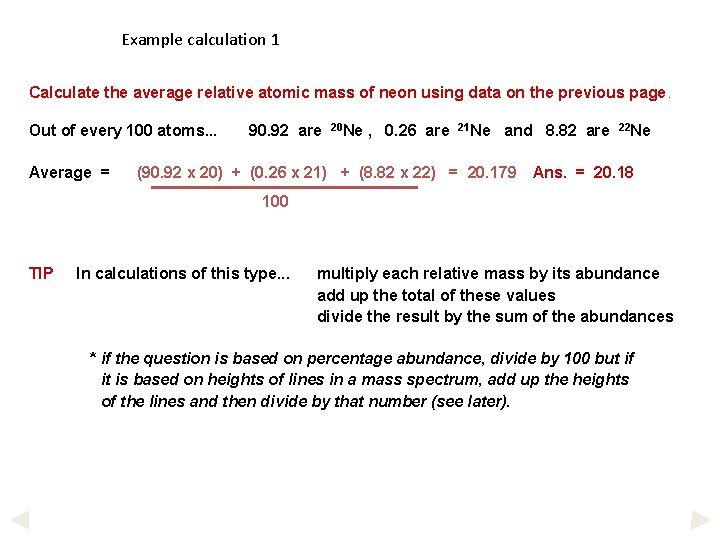Example calculation 1 Calculate the average relative atomic mass of neon using data on the previous page. Out of every 100 atoms. . . Average = 90. 92 are 20 Ne , 0. 26 are 21 Ne and 8. 82 are (90. 92 x 20) + (0. 26 x 21) + (8. 82 x 22) = 20. 179 22 Ne Ans. = 20. 18 100 TIP In calculations of this type. . . multiply each relative mass by its abundance add up the total of these values divide the result by the sum of the abundances * if the question is based on percentage abundance, divide by 100 but if it is based on heights of lines in a mass spectrum, add up the heights of the lines and then divide by that number (see later).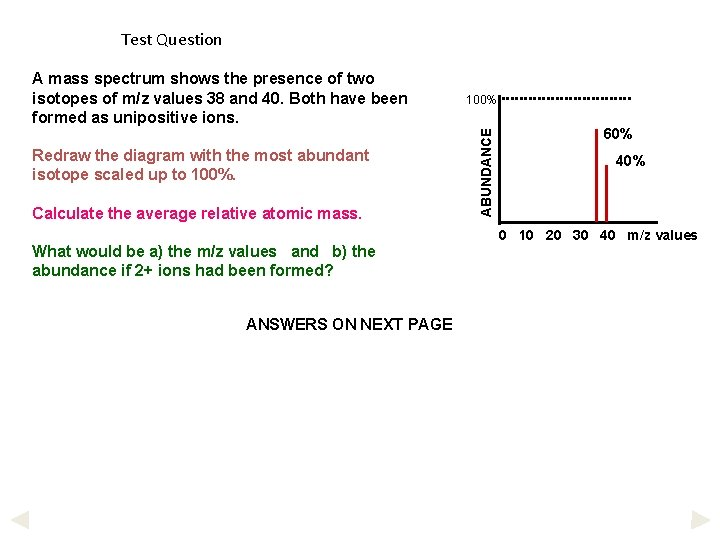Test Question Redraw the diagram with the most abundant isotope scaled up to 100%. Calculate the average relative atomic mass. What would be a) the m/z values and b) the abundance if 2+ ions had been formed? ANSWERS ON NEXT PAGE 100% ABUNDANCE A mass spectrum shows the presence of two isotopes of m/z values 38 and 40. Both have been formed as unipositive ions. 60% 40% 0 10 20 30 40 m/z values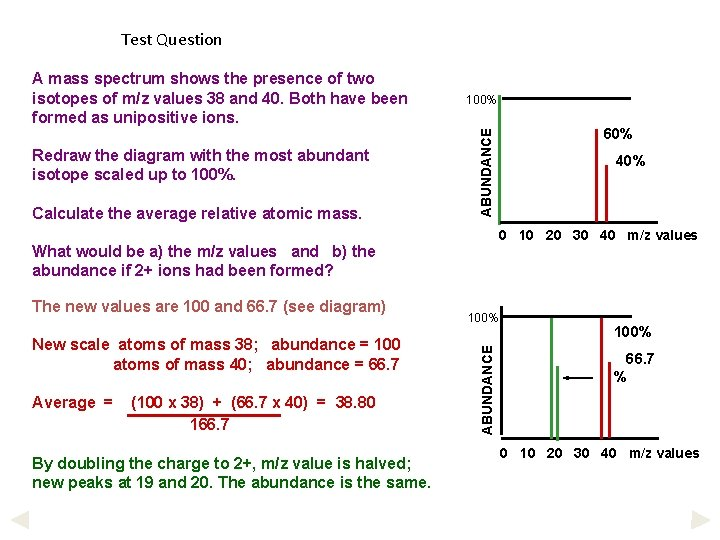Test Question Redraw the diagram with the most abundant isotope scaled up to 100%. Calculate the average relative atomic mass. 100% ABUNDANCE A mass spectrum shows the presence of two isotopes of m/z values 38 and 40. Both have been formed as unipositive ions. Average = (100 x 38) + (66. 7 x 40) = 38. 80 166. 7 By doubling the charge to 2+, m/z value is halved; new peaks at 19 and 20. The abundance is the same. 100% ABUNDANCE New scale atoms of mass 38; abundance = 100 atoms of mass 40; abundance = 66. 7 40% 0 10 20 30 40 m/z values What would be a) the m/z values and b) the abundance if 2+ ions had been formed? The new values are 100 and 66. 7 (see diagram) 60% 100% 66. 7 % 0 10 20 30 40 m/z valuesNow try these…. • Now give the questions in the textbook a go on your own (page 14)